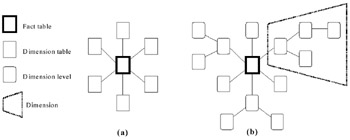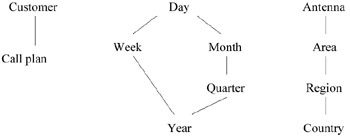# BACKGROUND

In this section we review the main terms and concepts related to multidimensional data. We first give some fundamental definitions, then introduce an example, which is used throughout the chapter to illustrate several issues related to multidimensional querying.

### Basic Definitions

Raw data (microdata). Multidimensional data are obtained by applying aggregations and statistical analysis functions over elementary data, usually called raw data or, with statistical terminology, microdata. The data representing each individual of a census survey, each customer call of a phone company, each medical treatment in a hospital, are possible examples of raw data. In most cases raw data are the product of complex activities of source integration, which are covered in detail in Chapter 12. Particularly, data have to be cleaned and reduced to a common integrated schema, solving possible conflicts in terms of both schema and contents.

Classification. An important issue in the production of raw data is that of classifying the properties of data. In this way, possibly continuous values are associated with a finite number of values, corresponding to the maximum degree of detail, by which each property of data is going to be analyzed. For example, in census data the birth date is transformed to an age in years, in hospitalization data a specific disease is classified and transformed into a(n) (alpha-)numerical code according to an international classification table.

Aggregation/grouping. As mentioned above, multidimensional data are obtained by applying aggregations and statistical functions on raw data. For example the datum "Number of inhabitants in Italy in 2001" is obtained by considering the set of microdata collected by the Italian 2001 Census and then applying a Count function on this set. Hence, an aggregation is basically a function mapping sets (or—in some cases—multisets, i.e., sets with duplicated elements) to numerical values. In multidimensional databases, aggregations are rarely applied on the whole set of microdata, but rather on several groups of data, each containing a subset of the data, homogeneous with respect to a given set of attributes.

For example, the data "Average duration of calls in 2001 by region and call plan" is obtained from the raw data corresponding to the phone calls in that year. Several groups are defined, each consisting of calls made in the same region and having the same call plan, and finally applying the average aggregation function on the duration attribute of the data in each group. The pair of values (region, call plan) is used to identify each group and associated with the corresponding average duration value. In multidimensional databases the attributes used to group data define the dimensions (in statistical terminology the category attributes), whereas the aggregate values the measures (in statistical terminology the summary attributes) of data.

Fact table. In many contexts the fact table coincides with the table of raw data. However, many authors usually define the fact table as constituted by dimension codes and measures, thus assuming that some form of classification and pre-aggregation on the data has been applied. Generally speaking, the fact table is the table comprising the measures of interest and the dimensions at the finest (allowed for querying) level of granularity.

Dimensions and dimension hierarchies. The term multidimensional data is due to the well-known metaphor of the data cube: for each of the n attributes, which is used to identify a single measure, a dimension of an n-dimensional space is considered. The possible values of the identifying attributes are mapped to points on the axis of the dimension, and in this way each point of this n-dimensional space is mapped to a single combination of the identifying attribute values and hence to a single aggregate value. The collection of all these points, along with all possible projections in lower dimensional spaces, constitutes the so-called data cube. In most cases, dimensions are structured in hierarchies, representing several levels of granularity of the corresponding measures. Hence a time dimension can be organized in days, months, and years; a territorial dimension in towns, regions, and countries; a product dimension in brands, families, and types.

When querying multidimensional data, the user specifies the measures of interest and the detail of the required information, by indicating for each dimension the desired level in the hierarchy. Note also that in a multidimensional environment querying is often an exploratory process, where the user "moves" along the dimension hierarchies, by increasing or reducing the granularity of displayed data. The operation of drill-down corresponds to increase the detail of data, e.g., by requesting the number of calls by region and month, starting from data about the number of calls by region and year, or from data about the number of calls by region. Conversely, the operation of roll-up allows the user to view data at a coarser level of granularity.

Star and snowflake schemes. In relational OLAP systems (also called ROLAP systems), facts and dimensions are usually mapped to (relational) tables: a central fact table and n surrounding dimension tables. This typical structure for multidimensional data is called star scheme and depicted in Figure 1(a). In star schemes dimension hierarchies are flattened (de-normalized) to minimize the number of joins required to express the queries and retrieve the data. In contrast, snowflake schemes (Figure 1(b)) use a normalized version of dimensions where each level in a hierarchy is associated with a distinct table. Snowflake schemes offer a more precise way to describe dimension hierarchies, but make the queries more complex to express, since they often require long sequences of join conditions, even for conceptually simple requests.Figure 1: Star and Snowflake Schemes

ROLAP and MOLAP systems. As shown above, ROLAP systems use conventional relational tables to store fact tables. The schema of a fact table is constituted by n dimensional attributes (n being the number of dimensions) and m measure attributes, where m is the number of measures stored in the fact table. The collection of dimensional attributes constitutes the primary key of the fact table, hence each m-tuple of measures is identified by a combination of n dimensional values. In contrast, for the storage of the fact table, MOLAP systems use multidimensional arrays coupled with (often proprietary) techniques of clustering and compression. In this way the fact table is stored as a consecutive sequence of values, where the meaning of each value is defined by the position of the value in the sequence. This results in lower space occupation, and it has been exploited to achieve better performances in the retrieval process and query evaluation.

### Running Example

We introduce an example, which is used throughout the chapter to illustrate the various issues related to multidimensional querying. A mobile phone company maintains a data warehouse containing the details on the traffic of its customers, in particular a table of raw data Calls on the schema:

`     (Id_call, Customer_no, Time, Ant_conn, Dur) `

where:

• Id_call is an identifier of the phone call.

• Customer_no is the phone number of the calling customer.

• Time is the time (day/hour/minute/second), when the call started.

• Ant_conn is the antenna to which the calling customer was connected when the call started.

• Dur is the duration (in seconds) of the call.

The table Calls could be considered a fact table, where Dur is the measure and the other attributes (except id_call) are the dimensions. In practice, however, analyses are made at a level of detail that does not require a precision of seconds for the Time dimension and an additional measure is useful, representing the number of calls. We therefore pre-aggregate the Calls table into the fact table Fcalls on the schema:

`     (Customer, Time, Ant_conn, Dur_sum, No_of_calls) `

where time has a maximum detail of days and Dur_sum is obtained by summing the duration of all calls for the same customer, day and antenna.

A tuple [c,t,a,d,n] on Fcalls represents the fact that there were n calls of global duration d made by customer c connected to antenna a in the day t. The table Fcalls can be obtained from Calls by first building groups of tuples corresponding to the same customer, day, and antenna, then by applying the aggregation functions Sum (on the duration attribute) and Count on each group, and finally by associating the combination of values for customer, day, and antenna, which identifies the group, to the two computed aggregation values. The dimensions of Fcalls are Customer, Time, and Antenna, and we suppose to define the hierarchies depicted in Figure 2 on them.Figure 2: The Dimension Hierarchies for Fcalls

In star schemes this corresponds to only three dimension tables, while in snowflake schema we would obtain 11 tables, one for each distinct dimension level. Typical queries on Fcalls may be: "Give me the global duration of calls by call plan, month, and region"; "Give me the number of calls for the first quarter of 2001 by call plan and area"; "Give me the average duration of calls in 2001 by region of the connecting antenna."Multidimensional Databases: Problems and Solutions
ISBN: 1591400538
EAN: 2147483647
Year: 2003
Pages: 150